# US7889437B2 - Zoom lens and image pickup apparatus having zoom lens - Google Patents

Zoom lens and image pickup apparatus having zoom lens Download PDF

## Info

Publication number
US7889437B2
US7889437B2 US12/544,759 US54475909A US7889437B2 US 7889437 B2 US7889437 B2 US 7889437B2 US 54475909 A US54475909 A US 54475909A US 7889437 B2 US7889437 B2 US 7889437B2
Authority
US
United States
Prior art keywords
lens
zoom
lens unit
zoom lens
unit
Prior art date
Legal status (The legal status is an assumption and is not a legal conclusion. Google has not performed a legal analysis and makes no representation as to the accuracy of the status listed.)
Active
Application number
US12/544,759
Other versions
US20090310228A1 (en
Inventor
Kenji Shinohara
Current Assignee (The listed assignees may be inaccurate. Google has not performed a legal analysis and makes no representation or warranty as to the accuracy of the list.)
Canon Inc
Original Assignee
Canon Inc
Priority date (The priority date is an assumption and is not a legal conclusion. Google has not performed a legal analysis and makes no representation as to the accuracy of the date listed.)
Filing date
Publication date
Priority to JP2007-203962 priority Critical
Priority to JP2007203962A priority patent/JP5053750B2/en
Priority to US12/186,399 priority patent/US7630144B2/en
Application filed by Canon Inc filed Critical Canon Inc
Priority to US12/544,759 priority patent/US7889437B2/en
Publication of US20090310228A1 publication Critical patent/US20090310228A1/en
Application granted granted Critical
Publication of US7889437B2 publication Critical patent/US7889437B2/en
Active legal-status Critical Current
Anticipated expiration legal-status Critical

## Links

• 230000003287 optical Effects 0.000 claims description 35
• 230000004075 alteration Effects 0.000 description 43
• 238000010586 diagram Methods 0.000 description 22
• 230000014509 gene expression Effects 0.000 description 22
• 238000003384 imaging method Methods 0.000 description 7
• 239000006185 dispersion Substances 0.000 description 6
• 239000000463 material Substances 0.000 description 5
• 230000000694 effects Effects 0.000 description 4
• 230000005499 meniscus Effects 0.000 description 4
• 238000006243 chemical reaction Methods 0.000 description 3
• 230000000875 corresponding Effects 0.000 description 3
• 238000001228 spectrum Methods 0.000 description 3
• 210000001747 Pupil Anatomy 0.000 description 1
• 201000009310 astigmatism Diseases 0.000 description 1
• 230000000295 complement Effects 0.000 description 1
• 230000003247 decreasing Effects 0.000 description 1
• 238000006073 displacement reaction Methods 0.000 description 1
• 239000004973 liquid crystal related substance Substances 0.000 description 1
• 229910044991 metal oxide Inorganic materials 0.000 description 1
• 150000004706 metal oxides Chemical class 0.000 description 1
• 230000004048 modification Effects 0.000 description 1
• 238000006011 modification reaction Methods 0.000 description 1
• 239000004065 semiconductor Substances 0.000 description 1
• 239000007787 solid Substances 0.000 description 1

## Images

•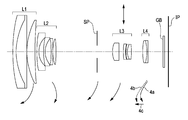•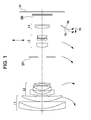•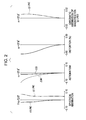•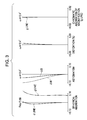•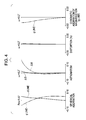•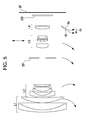•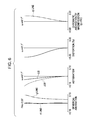•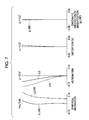•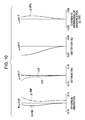•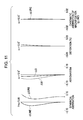•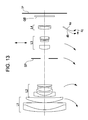•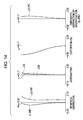•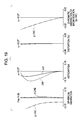•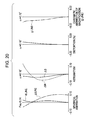•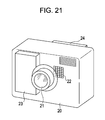## Classifications

• GPHYSICS
• G02OPTICS
• G02BOPTICAL ELEMENTS, SYSTEMS, OR APPARATUS
• G02B27/00Optical systems or apparatus not provided for by any of the groups G02B1/00 - G02B26/00, G02B30/00
• G02B27/64Imaging systems using optical elements for stabilisation of the lateral and angular position of the image
• G02B27/646Imaging systems using optical elements for stabilisation of the lateral and angular position of the image compensating for small deviations, e.g. due to vibration or shake
• GPHYSICS
• G02OPTICS
• G02BOPTICAL ELEMENTS, SYSTEMS, OR APPARATUS
• G02B15/00Optical objectives with means for varying the magnification
• G02B15/14Optical objectives with means for varying the magnification by axial movement of one or more lenses or groups of lenses relative to the image plane for continuously varying the equivalent focal length of the objective
• G02B15/144Optical objectives with means for varying the magnification by axial movement of one or more lenses or groups of lenses relative to the image plane for continuously varying the equivalent focal length of the objective having four groups only
• G02B15/1441Optical objectives with means for varying the magnification by axial movement of one or more lenses or groups of lenses relative to the image plane for continuously varying the equivalent focal length of the objective having four groups only the first group being positive
• G02B15/144113Optical objectives with means for varying the magnification by axial movement of one or more lenses or groups of lenses relative to the image plane for continuously varying the equivalent focal length of the objective having four groups only the first group being positive arranged +-++
• GPHYSICS
• G02OPTICS
• G02BOPTICAL ELEMENTS, SYSTEMS, OR APPARATUS
• G02B15/00Optical objectives with means for varying the magnification
• G02B15/14Optical objectives with means for varying the magnification by axial movement of one or more lenses or groups of lenses relative to the image plane for continuously varying the equivalent focal length of the objective
• G02B15/16Optical objectives with means for varying the magnification by axial movement of one or more lenses or groups of lenses relative to the image plane for continuously varying the equivalent focal length of the objective with interdependent non-linearly related movements between one lens or lens group, and another lens or lens group
• G02B15/163Optical objectives with means for varying the magnification by axial movement of one or more lenses or groups of lenses relative to the image plane for continuously varying the equivalent focal length of the objective with interdependent non-linearly related movements between one lens or lens group, and another lens or lens group having a first movable lens or lens group and a second movable lens or lens group, both in front of a fixed lens or lens group
• G02B15/167Optical objectives with means for varying the magnification by axial movement of one or more lenses or groups of lenses relative to the image plane for continuously varying the equivalent focal length of the objective with interdependent non-linearly related movements between one lens or lens group, and another lens or lens group having a first movable lens or lens group and a second movable lens or lens group, both in front of a fixed lens or lens group having an additional fixed front lens or group of lenses
• G02B15/173Optical objectives with means for varying the magnification by axial movement of one or more lenses or groups of lenses relative to the image plane for continuously varying the equivalent focal length of the objective with interdependent non-linearly related movements between one lens or lens group, and another lens or lens group having a first movable lens or lens group and a second movable lens or lens group, both in front of a fixed lens or lens group having an additional fixed front lens or group of lenses arranged +-+

## Abstract

A zoom lens includes, in order from an object side to an image side, first through fourth lens units. The first lens unit has positive refractive power, and the second lens unit has negative refractive power. The third lens unit has positive refractive power, the third lens unit having at least one positive lens and a plurality of negative lenses that include, in order from the object side to the image side, a first positive lens, a first negative lens, and a second negative lens. The fourth lens unit has positive refractive power. The zoom lens performs zooming by changing the distance between the lens units. The first positive lens is a positive lens closest to the object side among the at least one positive lens. The first negative lens is a negative lens closest to the object side among the plurality of negative lenses.

## Description

CROSS-REFERENCE TO RELATED APPLICATIONS
This application is a continuation of U.S. patent application Ser. No. 12/186,399 filed Aug. 5, 2008, which claims the benefit of Japanese Application No. 2007-203962 filed Aug. 6, 2007, all of which are hereby incorporated by reference herein in their entirety.
BACKGROUND OF THE INVENTION
1. Field of the Invention
The present invention relates to zoom lenses and image pickup apparatuses having the zoom lenses. The zoom lenses are suitably used in, for example, video cameras, electronic still cameras, broadcast cameras, silver-halide film cameras, and the like.
2. Description of the Related Art
Image pickup apparatuses these days, such as video cameras, digital still cameras, and broadcast cameras, which use solid-state image pickup elements, and silver-halide film cameras, have high performance and compact bodies. There are demands for short, compact, high-resolution zoom lenses that serve as imaging optical systems used with these image pickup apparatuses.
In addition, the zoom lenses are expected to have wide field angles and high zoom ratios.
An example of the zoom lens that meets the above-described demands is a four-unit zoom lens, which has four lens units, namely, in order from the object side to the image side, a first lens unit having positive refractive power, a second lens unit having negative refractive power, a third lens unit having positive refractive power, and a fourth lens unit having positive refractive power.
U.S. Pat. No. 7,193,790, and Japanese Patent Laid-Open No. 2006-113453 disclose rear-focusing four-unit zoom lenses that perform zooming by moving the first, second, and third lens units and correct image plane variation caused by zooming by moving the fourth lens unit.
United States Patent Application Publication No. 2007/0091460 discloses a rear-focusing four-unit zoom lens that vibrates the entire third lens unit in a direction perpendicular to the optical axis to form a still image.
In general, the size of a zoom lens can be reduced by increasing the refractive power of the lens units constituting the zoom lens and reducing the number of lenses.
However, in such a zoom lens, as the refractive power of the surfaces is increased, the thickness of the lenses is also increased. This makes it difficult to sufficiently reduce the length of the zoom lens or correct aberrations.
To obtain high zoom ratio and compact rear-focusing four-unit zoom lenses having high optical performance, it is important to appropriately set the refractive power, configuration, and the like of the lens units.
In particular, unless the refractive power of the third and fourth lens units as well as the configuration of the third lens unit are appropriately set, it is very difficult to produce rear-focusing four-unit zoom lenses having high zoom ratio and high optical performance over the entire zoom range.
SUMMARY OF THE INVENTION
The present invention is directed to a zoom lens and an image pickup apparatus including the zoom lens. According to an aspect of the present invention, a zoom lens includes, in order from an object side to an image side: a first lens unit having positive refractive power; a second lens unit having negative refractive power; a third lens unit having positive refractive power, the third lens unit having at least one positive lens and a plurality of negative lenses that include, in order from the object side to the image side, a first positive lens, a first negative lens, and a second negative lens; and a fourth lens unit having positive refractive power. The zoom lens performs zooming by changing the distance between the lens units. The first positive lens is a positive lens closest to the object side among the at least one positive lens. The first negative lens is a negative lens closest to the object side among the plurality of negative lenses. The zoom lens satisfies the following conditions:
0.20<Da3/TD3<0.35
0.3<f3/f4<1.0
where f3 is the focal length of the third lens unit, f4 is the focal length of the fourth lens unit, TD3 is the distance between the surface closest to the object side and the surface closest to the image side in the third lens unit, and Da3 is the distance between the surface of the first positive lens facing the image side and the surface of the first negative lens facing the object side.
Further features of the present invention will become apparent from the following description of exemplary embodiments with reference to the attached drawings.
BRIEF DESCRIPTION OF THE DRAWINGS
FIG. 1 is a sectional view of a zoom lens according to a first embodiment of the present invention, when set at the wide-angle end.
FIG. 2 shows aberration diagrams of the zoom lens according to the first embodiment (Numerical Example 1) of the present invention, when set at the wide-angle end.
FIG. 3 shows aberration diagrams of the zoom lens according to the first embodiment (Numerical Example 1) of the present invention, when set at a middle zooming position.
FIG. 4 is shows aberration diagrams of the zoom lens according to the first embodiment (Numerical Example 1) of the present invention, when set at the telephoto end.
FIG. 5 is a sectional view of a zoom lens according to a second embodiment of the present invention, when set at the wide-angle end.
FIG. 6 shows aberration diagrams of the zoom lens according to the second embodiment (Numerical Example 2) of the present invention, when set at the wide-angle end.
FIG. 7 shows aberration diagrams of the zoom lens according to the second embodiment (Numerical Example 2) of the present invention, when set at a middle zooming position.
FIG. 8 shows aberration diagrams of the zoom lens according to the second embodiment (Numerical Example 2) of the present invention, when set at the telephoto end.
FIG. 9 is a sectional view of a zoom lens according to a third embodiment of the present invention, when set at the wide-angle end.
FIG. 10 shows aberration diagrams of the zoom lens according to the third embodiment (Numerical Example 3) of the present invention, when set at the wide-angle end.
FIG. 11 shows aberration diagrams of the zoom lens according to the third embodiment (Numerical Example 3) of the present invention, when set at a middle zooming position.
FIG. 12 shows aberration diagrams of the zoom lens according to the third embodiment (Numerical Example 3) of the present invention, when set at the telephoto end.
FIG. 13 is a sectional view of a zoom lens according to a fourth embodiment of the present invention, when set at the wide-angle end.
FIG. 14 shows aberration diagrams of the zoom lens according to the fourth embodiment (Numerical Example 4) of the present invention, when set at the wide-angle end.
FIG. 15 shows aberration diagrams of the zoom lens according to the fourth embodiment (Numerical Example 4) of the present invention, when set at a middle zooming position.
FIG. 16 shows aberration diagrams of the zoom lens according to the fourth embodiment (Numerical Example 4) of the present invention, when set at the telephoto end.
FIG. 17 is a sectional view of a zoom lens according to a fifth embodiment of the present invention, when set at the wide-angle end.
FIG. 18 shows aberration diagrams of the zoom lens according to the fifth embodiment (Numerical Example 5) of the present invention, when set at the wide-angle end.
FIG. 19 shows aberration diagrams of the zoom lens according to the fifth embodiment (Numerical Example 5) of the present invention, when set at a middle zooming position.
FIG. 20 shows aberration diagrams of the zoom lens according to the fifth embodiment (Numerical Example 5) of the present invention, when set at the telephoto end.
FIG. 21 is a schematic view of an image pickup apparatus of the present invention.
DESCRIPTION OF THE EMBODIMENTS
The present invention provides a compact zoom lens having high optical performance over the entire zoom range, and an image pickup apparatus having the zoom lens. The zoom lens and the image pickup apparatus having the zoom lens according to embodiments of the present invention will be described.
The zoom lens according to the embodiments has, in order from the object side to the image side, a first lens unit having positive refractive power, a second lens unit having negative refractive power, a third lens unit having positive refractive power, and a fourth lens unit having positive refractive power. The zoom lens performs zooming by changing the distances between the lens units (the distance between the first and second lens units, the distance between the second and third lens units, and the distance between the third and fourth lens units). The zoom lens performs zooming by moving all the lens units, namely, the first, second, third, and fourth lens units. However, as long as the distances between the lens units are changed, it is not necessary to move all the lens units. For example, the zoom lens can perform zooming by changing only the first, second, and third lens units, or, only the second, third, and fourth lens units.
FIG. 1 is a sectional view of a zoom lens according to a first embodiment of the present invention, when set at the wide-angle end (short focal length).
FIGS. 2, 3, and 4 each show aberration diagrams of the zoom lens according to the first embodiment, when set at the wide-angle end, the middle zooming position, and the telephoto end (long focal length), respectively.
FIG. 5 is a sectional view of a zoom lens according to a second embodiment of the present invention, when set at the wide-angle end. FIGS. 6, 7, and 8 each show aberration diagrams of the zoom lens according to the second embodiment, when set at the wide-angle end, the middle zooming position, and the telephoto end, respectively.
FIG. 9 is a sectional view of a zoom lens according to a third embodiment of the present invention, when set at the wide-angle end. FIGS. 10, 11, and 12 each show aberration diagrams of the zoom lens according to the third embodiment, when set at the wide-angle end, the middle zooming position, and the telephoto end, respectively.
FIG. 13 is a sectional view of a zoom lens according to a fourth embodiment of the present invention, when set at the wide-angle end. FIGS. 14, 15, and 16 each show aberration diagrams of the zoom lens according to the fourth embodiment, when set at the wide-angle end, the middle zooming position, and the telephoto end, respectively.
FIG. 17 is a sectional view of a zoom lens according to a fifth embodiment of the present invention, when set at the wide-angle end. FIGS. 18, 19, and 20 each show aberration diagrams of the zoom lens according to the fifth embodiment, when set at the wide-angle end, the middle zooming position, and the telephoto end, respectively.
FIG. 21 is a schematic view of a relevant part of a camera (image pickup apparatus) having the zoom lens of the present invention. The zoom lens according to the embodiments is an imaging optical system used with an image pickup apparatus, such as a video camera, a digital camera, or a silver-halide film camera.
In the sectional views of the zoom lens, left side corresponds to the object side (front side), right side corresponds to the image side (rear side), i is the lens unit number counted from the object side, and Li is the i-th lens unit.
The sectional views of the zoom lens each show a first lens unit L1 having positive refractive power or optical power, which is the reciprocal of the focal length, a second lens unit L2 having negative refractive power, a third lens unit L3 having positive refractive power, and a fourth lens unit L4 having positive refractive power.
An aperture stop SP is located to the object side of the third lens unit L3. An optical block GB corresponds to an optical filter, a face plate, a crystal optical low-pass filter, or an infrared cut filter.
When the zoom lens is used as the imaging optical system of a video camera or a digital still camera, an image plane IP corresponds to the image pickup area of a solid-state image pickup element (photoelectric conversion element), such as a charge-coupled device (CCD) sensor or a complementary metal-oxide semiconductor (CMOS) sensor. When the zoom lens is used as the imaging optical system of a silver-halide film camera, the image plane IP corresponds to the photosurface, i.e., the film surface.
The aberration diagrams show the spherical aberrations with respect to the d line and the g line, the astigmatisms on the meridional image plane ΔM and the sagittal image plane ΔS, and the chromatic aberration of magnification with respect to the g line. In the aberration diagrams, ω is the half field angle, and Fno is the F number.
In the following embodiments, the terms “wide-angle end” and “telephoto end” refer to the zooming positions in which the lens units for zooming are positioned at both ends of the optical axis along which the lens unit can mechanically move.
In the embodiments, when the zoom lens zooms from the wide-angle end to the telephoto end, the lens units and the aperture stop SP are moved as indicated by the arrows.
More specifically, during zooming, the first lens unit L1 moves such that the position of the first lens unit L1 at the telephoto end is closer to the object side than the position of the first lens unit L1 at the wide-angle end.
The second lens unit L2 is moved along a locus convex toward the image side. The third lens unit L3 is moved toward the object side. The fourth lens unit L4 is moved along a locus convex toward the object side. The aperture stop SP is moved toward the object side independently of the lens units.
When the zoom lens zooms from the wide-angle end to the telephoto end, the first lens unit L1 and the third lens unit L3 are move toward the object side. This provides the zoom lens with a large zoom ratio while maintaining the compactness in length of the zoom lens when set at the wide-angle end.
In particular, in the embodiments, when the zoom lens performs zooming, the third lens unit L3 is moved toward the object side. This allows the third lens unit L3 and the fourth lens unit L4 to share the zooming ratio therebetween. Further, the first lens unit having positive refractive power L1 is moved toward the object side. This allows the second lens unit L2 to produce a great zooming effect. Thus, a high zoom ratio is provided without largely increasing the refractive powers of the first lens unit L1 and the second lens unit L2.
The zoom lens according to the embodiments is of a rear-focusing type, in which focusing is performed by moving the fourth lens unit L4 along the optical axis.
When the zoom lens set at the telephoto end shifts focus from an object at infinity to an object at a short distance, the fourth lens unit L4 is moved toward the front side as indicated by the arrow 4 c in the sectional views of the zoom lens. The solid curved arrow 4 a indicates the locus of movement of the fourth lens unit L4 for correcting image plane variation that occurs when the zoom lens focused on an object at infinity zooms from the wide-angle end to the telephoto end. The dashed curved arrow 4 b indicates the locus of movement of the fourth lens unit L4 for correcting image plane variation that occurs when the zoom lens focused on an object at a short distance zooms from the wide-angle end to the telephoto end.
As shown, by moving the fourth lens unit L4 along a locus convex toward the object side, the space between the third lens unit L3 and the fourth lens unit L4 is effectively utilized, whereby the total length of the zoom lens can be effectively reduced.
In the embodiments, the fourth lens unit L4, which is light in weight, is moved for focusing so as to allow the zoom lens to easily perform quick focusing and, for example, quick auto focusing.
Although the first lens unit L1 does not move in the optical axis direction for focusing, it may be moved independently or together with the fourth lens unit L4 if necessary for correcting aberrations.
In the embodiments, the entire or a part (the three lenses excluding the lens closest to the object side, or the cemented lens) of the third lens unit L3 is moved in a direction having a component perpendicular to the optical axis in order to correct the position of a captured image when the entire zoom lens is shaken. In other words, image blur (variation in image plane position on the image pickup element) is corrected by changing the position of the image of a subject.
In the embodiments, the aperture stop SP moves independently of the lens units when the zoom lens performs zooming. This enables the entrance pupil to be positioned on the object side in the wide-angle region, thereby preventing the diameter of the front lens (the effective diameter of the first lens unit) from increasing.
In the embodiments, the third lens unit L3 has a lens arrangement including, in order from the object side to the image side, a positive lens and a negative lens, and has at least two negative lenses in total. More specifically, the third lens unit L3 has, in order from the object side to the image side, a positive lens (a first positive lens), a negative lens (a first negative lens), a negative lens (a second negative lens), and a positive lens (a second positive lens). Alternatively, the third lens unit L3 may have, in order from the object side to the image side, a positive lens (a first positive lens), a negative lens (a first negative lens), a positive lens (a second positive lens), and a negative lens (a second negative lens).
The zoom lens of the present invention satisfies the following Conditional Expressions:
0.20<Da3/TD3<0.35  (1)
0.3<f3/f4<1.0  (2)
where f3 is the focal length of the third lens unit L3; f4 is the focal length of the fourth lens unit L4; TD3 is the distance between the surface closest to the object side and the surface closest to the image side in the third lens unit L3; and Da3 is the distance between the surface of the positive lens closest to the object side facing the image side and the surface of the negative lens closest to the object side facing the object side in the third lens unit L3.
Conditional Expression (1) concerns the total thickness of the third lens unit L3 (the distance between the lens surface closest to the object side and the lens surface closest to the image side) and the distance between the lenses in the third lens unit L3. If Da3/TD3 is smaller than the lower limit of Conditional Expression (1), the total thickness of the third lens unit L3 is small, which is advantageous in reducing the length of the zoom lens.
However, in order to enable the principal point to be positioned on the front side and to ensure a certain back focus, the thickness of the negative lens has to be increased. As a result, the weight of the third lens unit L3 serving as the optical image-stabilizing lens unit increases, which is undesirable.
On the other hand, if Da3/TD3 is larger than the upper limit of Conditional Expression (1), the total thickness of the third lens unit L3 is large. This increases the length of the zoom lens, which is also undesirable.
Conditional Expression (2) concerns the optical power ratio between the third lens unit L3 and the fourth lens unit L4. If f3/f4 is smaller than the lower limit of Conditional Expression (2), the power of the fourth lens unit L4 is small. This requires the fourth lens unit L4 to move a larger distance during focusing of the zoom lens, and consequently requires a larger space therefor. Thus, the length of the zoom lens increases. Further, variations of aberrations increase, which is also undesirable.
In addition, the amount by which the fourth lens unit L4 is moved to correct image plane variation during image-stabilizing operation increases. This increases variations of aberrations that occur during decentration, which is undesirable. On the other hand, if f3/f4 is larger than the upper limit of Conditional Expression (2), the power of the fourth lens unit L4 is large. This requires the fourth lens unit L4 to move a shorter distance during focusing of the zoom lens. However, the curvatures of the lens surfaces increase, and the number of lenses needs to be increased to suppress generation of aberrations in each lens surface. As a result, the length of the zoom lens increases, which is undesirable.
In the embodiments, in order to more effectively correct aberrations, minimize variations of aberrations that occur during zooming, and obtain high optical performance, it is desirable that the value ranges of Conditional Expressions (1) and (2) be set as follows:
0.20<Da3/TD3<0.33  (1a)
0.30<f3/f4<0.95  (2a)
It is further desirable that the value ranges of Conditional Expressions (1a) and (2a) be set as follows:
0.2<Da3/TD3<0.3  (1b)
0.3<f3/f4<0.9  (2b)
It is possible to provide a zoom lens having a high zoom ratio, compact size, and high performance, by setting the lens configuration as above.
In addition, it is desirable that the zoom lens according to the embodiments satisfy at least one of the following Conditional Expressions, so that the zoom lens can have advantages corresponding to the following Conditional Expressions:
−2.5<f32/f3<−0.8  (3)
10<νd3n<35  (4)
0.57<θgf3n<0.66  (5)
where f32 is the focal length of the second lens, which is a negative lens in the embodiments, of the third lens unit L3, counted from the object side; and νd3n and θgf3n are the Abbe number and the partial dispersion ratio, respectively, of the material of at least one of the two or more negative lenses that constitute the third lens unit L3.
The Abbe number νd and the partial dispersion ratio θgF are expressed as follows:
νd=(nd−1)/(nF−nC)
θgF=(nf−nF)/(nF−nC)
where ng, nF, nd, and nC are the refractive indices of the material for light with a wavelength of 435.8 nm (g line), light with a wavelength of 486.1 nm (F line), light with a wavelength of 587.6 nm (d line), and light with a wavelength of 656.3 nm (C line), respectively.
Conditional Expression (3) concerns the power distribution of the second negative lens of the third lens unit L3, counted from the object side. If f32/f3 is smaller than the lower limit of Conditional Expression (3), the power of the negative lens is small, and the power of the third lens unit L3 is large. Thus, curvature of field occurs on the under side.
On the other hand, if f32/f3 is larger than the upper limit of Conditional Expression (3), the power of the negative lens is large, and the power of the third lens unit L3 is small. This requires the third lens unit L3 to move a larger distance for zooming. Thus, the length of the zoom lens increases, which is undesirable.
Conditional Expressions (4) and (5) concern the lens material of the negative lens of the third lens unit L3. If νd3n is lower than the lower limit of Conditional Expression (4), the dispersion is large, and the power of the negative lens needs to be decreased to correct axial chromatic aberration. As a result, curvature of field occurs on the under side. On the other hand, if νd3n is higher than the upper limit of Conditional Expression (4), dispersion decreases and axial chromatic aberration is under-corrected.
If θgf3n is lower than the lower limit of Conditional Expression (5), the partial dispersion ratio is small, and the effect of correcting a secondary spectrum of axial chromatic aberration is large. However, such a large correction effect reduces the power of the negative lens, whereby curvature of field occurs on the under side.
On the other hand, if θgf3n is larger than the upper limit of Conditional Expression (5), the partial dispersion ratio is large, and the effect of correcting a secondary spectrum of axial chromatic aberration is small. In particular, the secondary spectrum is under-corrected when the zoom lens is set at a high zoom ratio and the telephoto end.
From the standpoint of aberration correction, it is more desirable that the value ranges of Conditional Expression (3) to (5) be set as follows:
−2.2<f32/f3<−0.9  (3a)
11<νd3n<34  (4a)
0.58<θgf3n<0.65  (5a)
It is further desirable that the value ranges of Conditional Expressions (3a), (4a), and (5a) be set as follows:
−2.00<f32/f3<−0.95  (3b)
18<νd3n<33  (4b)
0.59<θgf3n<0.64  (5b)
It is possible to provide a zoom lens having a compact size, simple lens configuration, and high optical performance over the entire zoom range and object distance range, by setting the lens configuration as above.
Now, the characteristics of the lens configuration of the lens units according to the embodiments will be described.
The first lens unit L1 has a larger effective lens diameter than the other lens units. Thus, it is desirable that the first lens unit L1 include a smaller number of lenses from the stand point of reducing weight.
The first lens unit L1 has at least one negative lens and two positive lenses.
More specifically, the first lens unit L1 has, in order from the object side to the image side, a cemented lens consisting of a meniscus negative lens with the convex surface facing the object side and a positive lens, and a positive lens. This configuration effectively corrects spherical aberration and chromatic aberration that frequently occur in a zoom lens having higher zoom ratio.
The second lens unit L2 has four independent lenses, namely, two meniscus negative lenses with the convex surfaces facing the object side, a negative lens with the concave surface facing the object side, and a positive lens with the convex surface facing the object side.
This configuration reduces variations of aberrations caused by zooming. In particular, this configuration effectively corrects distortion that occurs when the zoom lens is set at the wide-angle end, and spherical aberration that occurs when the zoom lens is set at the telephoto end.
The third lens unit L3 has, in order from the object side to the image side, a positive lens (a first positive lens) with the convex surface facing the object side, a meniscus negative lens (a first negative lens) with the convex surface facing the object side, and a cemented lens consisting of a meniscus negative lens (a second negative lens) with the convex surface facing the object side and a positive lens (a second positive lens).
The third lens unit L3 has one or more aspherical surfaces. This configuration effectively corrects variations of aberrations caused by zooming.
The use of the cemented lens in the third lens unit L3 serves to minimize variation of chromatic aberration caused by zooming. This also serves to minimize occurrence of aberrations caused by decentration during image-stabilizing operation, in which the entire or a part of the third lens unit L3 is subject to decentration in a direction having a component perpendicular to the optical axis.
The fourth lens unit L4 has a cemented lens consisting of a positive lens with the convex surface facing the object side and a negative lens.
Numerical Examples 1 to 5 corresponding to the first to fifth embodiments of the present invention are shown below. In the numerical examples, i is the optical surface number counted from the object side, Ri is the radius of curvature of the i-th optical surface (the i-th surface), Di is the distance between the i-th surface and the i+1-th surface, Ni is the refractive index of the material of the i-th optical component for the d line, and νi is the Abbe number of the material of the i-th optical component for the d line.
The shape of the aspherical surface is expressed as follows:
x=(h 2 /R)/[1+[1−(1+k)(h/R)2]1/2 ]+Bh 4 +Ch 6 +Dh 8 +Eh 10 +Fh 12 +Gh 14 +Hh 16
where k is the eccentricity; B, C, D, E, F, G, and H are the aspherical surface coefficients; x is the displacement from the surface vertex, in the optical axis direction and at the height h from the optical axis; and R is the radius of curvature. For example, e-Z means 10−Z. In addition, f is the focal length, Fno is the F number, and ω is the half field angle.
In the numerical examples, the last two surfaces are the surfaces of an optical block, such as a filter or a face plate.
Table 1 lists Conditional Expressions (1) to (5) and the corresponding values in Numerical Examples 1 to 5.
Numerical Example 1
 f = 1~13.71 Fno = 2.89~5.57 2ω = 75.2°~6.4°
 R1 = 13.578 D1 = 0.33 N1 = 1.806100 ν1 = 33.3 R2 = 5.850 D2 = 1.02 N2 = 1.496999 ν2 = 81.5 R3 = 162.493 D3 = 0.03 R4 = 6.244 D4 = 0.76 N3 = 1.696797 ν3 = 55.5 R5 = 31.628 D5 = variable R6 = 9.845 D6 = 0.18 N4 = 1.882997 ν4 = 40.8 R7 = 1.798 D7 = 0.45 R8 = 6.219 D8 = 0.15 N5 = 1.882997 ν5 = 40.8 R9 = 1.859 D9 = 0.58 R10 = −4.443 D10 = 0.14 N6 = 1.806100 ν6 = 33.3 R11 = 58.969 D11 = 0.02 R12 = 4.038 D12 = 0.29 N7 = 1.922860 ν7 = 18.9 R13 = −14.018 D13 = variable R14 = stop D14 = variable R15* = 1.846 D15 = 0.62 N8 = 1.583126 ν8 = 59.4 R16 = 64.350 D16 = 0.41 R17 = 7.983 D17 = 0.21 N9 = 1.581439 ν9 = 40.8 R18 = 1.994 D18 = 0.05 R19 = 3.003 D19 = 0.14 N10 = 2.003300 ν10 = 28.3 R20 = 1.332 D20 = 0.39 N11 = 1.712995 ν11 = 53.9 R21 = −7.630 D21 = variable R22 = 4.487 D22 = 0.41 N12 = 1.754998 ν12 = 52.3 R23 = −3.750 D23 = 0.10 N13 = 1.834000 ν13 = 37.2 R24 = 19.645 D24 = variable R25 = ∞ D25 = 0.14 N14 = 1.516330 ν14 = 64.1 R26 = ∞
 zoom ratio 13.71
 wide angle middle telephoto focal length 1.00 8.07 13.71 F number 2.87 3.88 5.57 field angle 37.6 12.4 3.2 image height 0.75 0.75 0.75 total length of lens 14.8 15.9 19.7 BF 1.87 3.08 1.79 D5 0.17 5.63 6.56 D13 3.92 0.45 0.26 D14 1.47 0.28 0.19 D21 1.07 1.99 4.61 D24 1.45 3.03 1.37
 unit first surface focal length 1 1 10.71 2 6 −1.68 3 15 3.32 4 22 9.29
 aspherical surface coefficient
 R16 k = −1.12605e−01 B = −1.29138e−02 C = 1.74008e−03 D = −8.76570e−03 E = 6.98905e−03 F = 0.00000e+00 G = 0.00000e+00 H = 0.00000e+00
Numerical Example 2
 f = 1~13.73 Fno = 2.87~5.50 2ω = 75.3°~6.4°
 R1 = 13.647 D1 = 0.33 N1 = 1.806100 ν1 = 33.3 R2 = 5.864 D2 = 1.02 N2 = 1.496999 ν2 = 81.5 R3 = 170.503 D3 = 0.03 R4 = 6.267 D4 = 0.76 N3 = 1.696797 ν3 = 55.5 R5 = 32.141 D5 = variable R6 = 9.863 D6 = 0.18 N4 = 1.882997 ν4 = 40.8 R7 = 1.793 D7 = 0.46 R8 = 6.243 D8 = 0.15 N5 = 1.882997 ν5 = 40.8 R9 = 1.862 D9 = 0.58 R10 = −4.514 D10 = 0.14 N6 = 1.806100 ν6 = 33.3 R11 = 50.598 D11 = 0.02 R12 = 3.975 D12 = 0.29 N7 = 1.922860 ν7 = 18.9 R13 = −13.670 D13 = variable R14 = stop D14 = variable R15* = 1.868 D15 = 0.62 N8 = 1.583126 ν8 = 59.4 R16 = 32.615 D16 = 0.41 R17 = 5.511 D17 = 0.21 N9 = 1.582673 ν9 = 46.4 R18 = 2.135 D18 = 0.04 R19 = 3.024 D19 = 0.14 N10 = 2.003300 ν10 = 28.3 R20 = 1.265 D20 = 0.39 N11 = 1.696797 ν11 = 55.5 R21 = −9.816 D21 = variable R22 = 4.511 D22 = 0.41 N12 = 1.754998 ν12 = 52.3 R23 = −4.113 D23 = 0.10 N13 = 1.834000 ν13 = 37.2 R24 = 27.588 D24 = variable R25 = ∞ D25 = 0.14 N14 = 1.516330 ν14 = 64.1 R26 = ∞
 zoom ratio 13.73
 wide angle middle telephoto focal length 1.00 7.96 13.73 F number 2.87 3.84 5.50 field angle 37.7 12.6 3.2 image height 0.75 0.75 0.75 total length of lens 14.8 16.0 19.7 BF 1.89 3.02 1.85 D5 0.17 5.65 6.60 D13 3.95 0.47 0.26 D14 1.48 0.28 0.19 D21 1.05 2.10 4.57 D24 1.45 2.91 1.40
 unit first surface focal length 1 1 10.72 2 6 −1.71 3 15 3.37 4 22 8.33
 aspherical surface coefficient
 R15 k = −6.27526e−02 B = −1.31808e−02 C = 1.82424e−03 D = −8.73109e−03 E = 6.90110e−03 F = 0.00000e+00 G = 0.00000e+00 H = 0.00000e+00
Numerical Example 3
 f = 1~13.72 Fno = 2.87~5.53 2ω = 75.3°~6.4°
 R1 = 13.538 D1 = 0.33 N1 = 1.806100 ν1 = 33.3 R2 = 5.876 D2 = 1.02 N2 = 1.496999 ν2 = 81.5 R3 = 174.059 D3 = 0.03 R4 = 6.224 D4 = 0.77 N3 = 1.696797 ν3 = 55.5 R5 = 31.540 D5 = variable R6 = 9.879 D6 = 0.18 N4 = 1.882997 ν4 = 40.8 R7 = 1.787 D7 = 0.46 R8 = 6.216 D8 = 0.15 N5 = 1.882997 ν5 = 40.8 R9 = 1.861 D9 = 0.59 R10 = −4.409 D10 = 0.14 N6 = 1.806100 ν6 = 33.3 R11 = 61.564 D11 = 0.02 R12 = 4.113 D12 = 0.37 N7 = 1.922860 ν7 = 18.9 R13 = −13.257 D13 = variable R14 = stop D14 = variable R15* = 1.837 D15 = 0.62 N8 = 1.583126 ν8 = 59.4 R16 = 60.337 D16 = 0.44 R17 = 8.527 D17 = 0.21 N9 = 1.595509 ν9 = 39.2 R18 = 1.974 D18 = 0.05 R19 = 3.006 D19 = 0.14 N10 = 2.003300 ν10 = 28.3 R20 = 1.370 D20 = 0.39 N11 = 1.712995 ν11 = 53.9 R21 = −7.728 D21 = variable R22 = 4.418 D22 = 0.41 N12 = 1.754998 ν12 = 52.3 R23 = −3.797 D23 = 0.10 N13 = 1.834000 ν13 = 37.2 R24 = 22.664 D24 = variable R25 = ∞ D25 = 0.14 N14 = 1.516330 ν14 = 64.1 R26 = ∞
 zoom ratio 13.72
 wide angle middle telephoto focal length 1.00 8.00 13.72 F number 2.87 3.85 5.53 field angle 37.7 12.6 3.2 image height 0.75 0.75 0.75 total length of lens 14.9 16.0 19.8 BF 1.91 3.08 1.83 D5 0.17 5.59 6.53 D13 3.84 0.44 0.26 D14 1.52 0.29 0.19 D21 1.04 2.04 4.58 D24 1.50 3.00 1.41
 unit first surface focal length 1 1 10.61 2 6 −1.68 3 15 3.37 4 22 8.63
 aspherical surface coefficient
 R15 k = −5.82469e−02 B = −1.38910e−02 C = 7.57297e−04 D = −8.91734e−03 E = 6.88889e−03 F = 0.00000e+00 G = 0.00000e+00 H = 0.00000e+00
Numerical Example 4
 f = 1~17.0 Fno = 2.87~5.74 2ω = 75.4°~5.1°
 R1 = 13.891 D1 = 0.33 N1 = 1.806100 ν1 = 33.3 R2 = 5.854 D2 = 1.03 N2 = 1.496999 ν2 = 81.5 R3 = 232.443 D3 = 0.03 R4 = 6.680 D4 = 0.76 N3 = 1.729157 ν3 = 54.7 R5 = 49.490 D5 = variable R6 = 12.500 D6 = 0.18 N4 = 1.882997 ν4 = 40.8 R7 = 1.810 D7 = 0.44 R8 = 5.862 D8 = 0.15 N5 = 1.882997 ν5 = 40.8 R9 = 1.872 D9 = 0.59 R10 = −4.349 D10 = 0.14 N6 = 1.806100 ν6 = 33.3 R11 = 51.393 D11 = 0.02 R12 = 4.005 D12 = 0.33 N7 = 1.922860 ν7 = 18.9 R13 = −13.683 D13 = variable R14 = stop D14 = variable R15* = 1.874 D15 = 0.62 N8 = 1.583126 ν8 = 59.4 R16 = 144.162 D16 = 0.52 R17 = 9.133 D17 = 0.21 N9 = 1.595509 ν9 = 39.2 R18 = 2.049 D18 = 0.04 R19 = 3.024 D19 = 0.14 N10 = 2.003300 ν10 = 28.3 R20 = 1.319 D20 = 0.39 N11 = 1.712995 ν11 = 53.9 R21 = −9.408 D21 = variable R22 = 3.978 D22 = 0.41 N12 = 1.772499 ν12 = 49.6 R23 = −4.003 D23 = 0.10 N13 = 1.834000 ν13 = 37.2 R24 = 25.603 D24 = variable R25 = ∞ D25 = 0.14 N14 = 1.516330 ν14 = 64.1 R26 = ∞
 zoom ratio 17.0
 wide angle middle telephoto focal length 1.00 8.40 17.00 F number 2.87 3.68 5.74 field angle 37.7 12.4 2.5 image height 0.75 0.75 0.75 total length of lens 15.0 16.1 20.4 BF 1.88 3.09 0.98 D5 0.17 5.66 6.57 D13 3.93 0.42 0.28 D14 1.42 0.23 0.23 D21 1.14 2.16 5.96 D24 1.44 2.93 0.54
 unit first surface focal length 1 1 10.26 2 6 −1.67 3 15 3.53 4 22 6.72
 aspherical surface coefficient
 R15 k = −1.28224e−01 B = −1.20097e−02 C = 2.31091e−03 D = −8.60297e−03 E = 5.31285e−03 F = 0.00000e+00 G = 0.00000e+00 H = 0.00000e+00
Numerical Example 5
 f = 1~20.5 Fno = 2.87~5.74 2ω = 75.4°~4.19°
 R1 = 13.689 D1 = 0.33 N1 = 1.806100 ν1 = 33.3 R2 = 5.772 D2 = 1.02 N2 = 1.496999 ν2 = 81.5 R3 = 286.543 D3 = 0.03 R4 = 6.590 D4 = 0.76 N3 = 1.691002 ν3 = 54.8 R5 = 57.149 D5 = variable R6 = 12.921 D6 = 0.18 N4 = 1.882997 ν4 = 40.8 R7 = 1.829 D7 = 0.44 R8 = 6.079 D8 = 0.15 N5 = 1.834807 ν5 = 42.7 R9 = 1.881 D9 = 0.58 R10 = −4.380 D10 = 0.14 N6 = 1.806100 ν6 = 33.3 R11 = 40.602 D11 = 0.02 R12 = 4.014 D12 = 0.28 N7 = 1.922860 ν7 = 18.9 R13 = −13.918 D13 = variable R14 = stop D14 = variable R15* = 1.821 D15 = 0.62 N8 = 1.583126 ν8 = 59.4 R16 = −19.233 D16 = 0.55 R17 = 18.158 D17 = 0.21 N9 = 1.605620 ν9 = 43.7 R18 = 2.016 D18 = 0.05 R19 = 3.308 D19 = 0.12 N10 = 2.003300 ν10 = 28.3 R20 = 1.299 D20 = 0.39 N11 = 1.712995 ν11 = 53.9 R21 = −13.296 D21 = variable R22 = 3.503 D22 = 0.41 N12 = 1.754998 ν12 = 52.3 R23 = −4.582 D23 = 0.10 N13 = 1.834000 ν13 = 37.2 R24 = 274.040 D24 = variable R25 = ∞ D25 = 0.14 N14 = 1.516330 ν14 = 64.1 R26 = ∞
 zoom ratio 20.5
 wide angle middle telephoto focal length 1.00 8.46 20.50 F number 2.87 4.20 5.74 field angle 37.7 5.07 2.10 image height 0.75 0.75 0.75 total length of lens 13.15 15.33 19.58 BF 1.94 3.45 1.23 D5 0.17 5.83 7.01 D13 3.98 0.49 0.26 D14 1.55 0.24 0.21 D21 1.08 2.38 5.72 D24 1.51 3.01 0.79
 unit first surface focal length 1 1 10.40 2 6 −1.69 3 15 3.80 4 22 5.09
 aspherical surface coefficient
 R15 k = −3.07164e−01 B = −1.04243e−02 C = 3.00760e−03 D = −9.93328e−03 E = 7.79403e−03 F = 0.00000e+00 G = 0.00000e+00 H = 0.00000e+00
 TABLE 1 Numerical Numerical Numerical Numerical Numerical Example 1 Example 2 Example 3 Example 4 Example 5
 (1) 0.23 0.23 0.24 0.27 0.28 (2) 0.36 0.41 0.39 0.52 0.75 (3) −1.40 −1.82 −1.29 −1.27 −0.99 (4) 28.3 28.3 28.3 28.3 28.3 (5) 0.598 0.598 0.598 0.598 0.598
A digital still camera according to an embodiment, which uses the zoom lens according to the above-described embodiments as the imaging optical system, will now be described with reference to FIG. 21.
FIG. 21 shows a camera body 20, an imaging optical system 21 that includes the zoom lens according to any one of the first to fifth embodiments, a solid-state image pickup element (photoelectric conversion element) 22, such as a CCD sensor or a CMOS sensor, which is built in the camera body and receives an image of a subject formed by the imaging optical system 21, a memory 23 that records information of the image of the subject after being subjected to photoelectric conversion by the solid-state image pickup element 22, and a viewfinder 24 that includes a liquid crystal display panel or the like for observing the image of the subject formed on the solid-state image pickup element 22.
It is possible to make a compact image pickup apparatus having high optical performance, by using the zoom lens of the present invention in an image pickup apparatus, such as a digital still camera.
As has been described, according to the above-described embodiments, it is possible to provide a compact zoom lens having high optical performance over the entire zoom range, and an image pickup apparatus having the zoom lens.
While the present invention has been described with reference to exemplary embodiments, it is to be understood that the invention is not limited to the disclosed exemplary embodiments. The scope of the following claims is to be accorded the broadest interpretation so as to encompass all modifications and equivalent structures and functions.

## Claims (7)

1. A zoom lens comprising, in order from an object side to an image side:
a first lens unit having positive refractive power;
a second lens unit having negative refractive power;
a third lens unit having positive refractive power, the third lens unit having, in order from the object side to the image side, a first positive lens, a first negative lens, and a second negative lens; and
a fourth lens unit having positive refractive power,
wherein the zoom lens performs zooming by changing the distance between the lens units, and
wherein the zoom lens satisfies the following conditions:

0.20<Da3/TD3<0.35

0.3<f3/f4<1.0
where f3 is the focal length of the third lens unit, f4 is the focal length of the fourth lens unit, TD3 is the distance between the surface closest to the object side and the surface closest to the image side in the third lens unit, and Da3 is the distance between the surface of the first positive lens facing the image side and the surface of the first negative lens facing the object side.
2. The zoom lens according to claim 1,
wherein the entire or a part of the third lens unit is moved in a direction having a component perpendicular to an optical axis to change the position of an image of a subject formed by the zoom lens.
3. The zoom lens according to claim 1,
wherein, when the zoom lens zooms from a wide-angle end to a telephoto end, the first lens unit moves such that the position of the first lens unit at the telephoto end is closer to the object side than the position of the first lens unit at the wide-angle end, the second lens unit moves along a locus convex toward the image side, the third lens unit moves toward the object side, and the fourth lens unit moves along a locus convex toward the object side.
4. The zoom lens according to claim 1, further comprising an aperture stop disposed between the second lens unit and the third lens unit, the aperture stop being moved in an optical axis direction when the zoom lens zooms from the wide-angle end to the telephoto end.
5. The zoom lens according to claim 1, wherein the zoom lens is configured to form an image of an object on a solid-state image pickup element.
6. The zoom lens according to claim 1,
wherein the first positive lens is a positive lens closest to the object side in the third lens unit, and
wherein the first negative lens is a negative lens closest to the object side in the third lens unit.
7. An image pickup apparatus comprising:
the zoom lens according to claim 1; and
a solid-state image pickup element configured to receive an image formed by the zoom lens.
US12/544,759 2007-08-06 2009-08-20 Zoom lens and image pickup apparatus having zoom lens Active US7889437B2 (en)

## Priority Applications (4)

Application Number Priority Date Filing Date Title
JP2007-203962 2007-08-06
JP2007203962A JP5053750B2 (en) 2007-08-06 2007-08-06 Zoom lens and imaging apparatus having the same
US12/186,399 US7630144B2 (en) 2007-08-06 2008-08-05 Zoom lens and image pickup apparatus having zoom lens
US12/544,759 US7889437B2 (en) 2007-08-06 2009-08-20 Zoom lens and image pickup apparatus having zoom lens

## Applications Claiming Priority (1)

Application Number Priority Date Filing Date Title
US12/544,759 US7889437B2 (en) 2007-08-06 2009-08-20 Zoom lens and image pickup apparatus having zoom lens

## Related Parent Applications (1)

Application Number Title Priority Date Filing Date
US12/186,399 Continuation US7630144B2 (en) 2007-08-06 2008-08-05 Zoom lens and image pickup apparatus having zoom lens

## Publications (2)

Publication Number Publication Date
US20090310228A1 US20090310228A1 (en) 2009-12-17
US7889437B2 true US7889437B2 (en) 2011-02-15

# Family

## Family Applications (2)

Application Number Title Priority Date Filing Date
US12/186,399 Active US7630144B2 (en) 2007-08-06 2008-08-05 Zoom lens and image pickup apparatus having zoom lens
US12/544,759 Active US7889437B2 (en) 2007-08-06 2009-08-20 Zoom lens and image pickup apparatus having zoom lens

## Family Applications Before (1)

Application Number Title Priority Date Filing Date
US12/186,399 Active US7630144B2 (en) 2007-08-06 2008-08-05 Zoom lens and image pickup apparatus having zoom lens

## Country Status (2)

Country Link
US (2) US7630144B2 (en)
JP (1) JP5053750B2 (en)

## Cited By (1)

* Cited by examiner, † Cited by third party
Publication number Priority date Publication date Assignee Title
US9513471B2 (en) 2010-03-08 2016-12-06 Nikon Corporation Zoom lens system, optical apparatus and method for manufacturing zoom lens system

## Families Citing this family (13)

* Cited by examiner, † Cited by third party
Publication number Priority date Publication date Assignee Title
JP5111007B2 (en) * 2007-08-06 2012-12-26 キヤノン株式会社 Zoom lens and imaging apparatus having the same
JP5072474B2 (en) * 2007-08-06 2012-11-14 キヤノン株式会社 Zoom lens and imaging apparatus having the same
JP5344549B2 (en) * 2008-08-08 2013-11-20 キヤノン株式会社 Zoom lens and imaging apparatus having the same
JP5241377B2 (en) * 2008-08-19 2013-07-17 キヤノン株式会社 Zoom lens and imaging apparatus having the same
JP5274265B2 (en) * 2009-01-07 2013-08-28 キヤノン株式会社 Zoom lens and imaging apparatus having the same
JP5460228B2 (en) * 2009-04-02 2014-04-02 キヤノン株式会社 Zoom lens and imaging apparatus having the same
JP5350163B2 (en) * 2009-10-02 2013-11-27 キヤノン株式会社 Image projection device
JPWO2011102091A1 (en) 2010-02-16 2013-06-17 パナソニック株式会社 Zoom lens system, imaging device and camera
JP5724189B2 (en) * 2010-03-08 2015-05-27 株式会社ニコン Variable magnification optical system, optical device
JP2012048033A (en) 2010-08-27 2012-03-08 Hoya Corp High zoom-ratio zoom lens system
JP5872791B2 (en) * 2011-05-02 2016-03-01 オリンパス株式会社 Zoom lens and image pickup apparatus including the same
JP5751991B2 (en) * 2011-08-30 2015-07-22 キヤノン株式会社 Zoom lens and imaging apparatus having the same
JP5906759B2 (en) 2012-01-25 2016-04-20 株式会社ニコン Zoom lens, optical device, and method of manufacturing zoom lens

## Citations (3)

* Cited by examiner, † Cited by third party
Publication number Priority date Publication date Assignee Title
US4629294A (en) * 1983-07-04 1986-12-16 Canon Kabushiki Kaisha Zoom lens
US5585966A (en) * 1993-12-28 1996-12-17 Nikon Corporation Zoom lens with vibration reduction function
US20020063961A1 (en) * 1997-03-18 2002-05-30 Hiroyuki Hamano Variable magnification optical system having image stabilizing function

## Family Cites Families (6)

* Cited by examiner, † Cited by third party
Publication number Priority date Publication date Assignee Title
JP3619178B2 (en) * 2001-09-28 2005-02-09 キヤノン株式会社 Zoom lens and optical apparatus having the same
JP4794845B2 (en) 2004-10-18 2011-10-19 キヤノン株式会社 Zoom lens and imaging apparatus having the same
JP3960334B2 (en) * 2004-12-20 2007-08-15 コニカミノルタフォトイメージング株式会社 Imaging optical system and imaging apparatus
JP4817699B2 (en) * 2005-04-01 2011-11-16 キヤノン株式会社 Zoom lens and imaging apparatus having the same
JP4301226B2 (en) * 2005-09-15 2009-07-22 コニカミノルタオプト株式会社 Variable magnification optical system and imaging apparatus
JP4847091B2 (en) * 2005-10-15 2011-12-28 キヤノン株式会社 Zoom lens and imaging apparatus having the same

## Patent Citations (3)

* Cited by examiner, † Cited by third party
Publication number Priority date Publication date Assignee Title
US4629294A (en) * 1983-07-04 1986-12-16 Canon Kabushiki Kaisha Zoom lens
US5585966A (en) * 1993-12-28 1996-12-17 Nikon Corporation Zoom lens with vibration reduction function
US20020063961A1 (en) * 1997-03-18 2002-05-30 Hiroyuki Hamano Variable magnification optical system having image stabilizing function

## Cited By (1)

* Cited by examiner, † Cited by third party
Publication number Priority date Publication date Assignee Title
US9513471B2 (en) 2010-03-08 2016-12-06 Nikon Corporation Zoom lens system, optical apparatus and method for manufacturing zoom lens system

## Also Published As

Publication number Publication date
JP5053750B2 (en) 2012-10-17
US20090040624A1 (en) 2009-02-12
US7630144B2 (en) 2009-12-08
JP2009042269A (en) 2009-02-26
US20090310228A1 (en) 2009-12-17

## Similar Documents

Publication Publication Date Title
US9952446B2 (en) Zoom lens and image pickup apparatus including the same
US8503095B2 (en) Zoom lens system and image pickup apparatus including the same
US6449433B2 (en) Taking lens device
US8988588B2 (en) Zoom lens and image pickup apparatus including the same
EP2124082B1 (en) Telephoto vibration-proof zoom lens having five lens groups and rear-focusing
US7268811B2 (en) Zoom lens system
US7139131B2 (en) Zoom lens system and image pickup apparatus having the same
JP4881035B2 (en) Zoom lens and imaging apparatus having the same
JP4323796B2 (en) Zoom lens and imaging apparatus having the same
US8351130B2 (en) Zoom lens and image pickup apparatus equipped with same
JP4315450B2 (en) Zoom lens system and imaging apparatus
US6404561B1 (en) Taking lens apparatus
US7982967B2 (en) Zoom lens and image pickup apparatus including the same
US7102829B2 (en) Zoom lens system and image pickup device having zoom lens system
US7471460B2 (en) Zoom lens system and camera having same
US7057818B2 (en) Zoom lens and optical apparatus having the same
US7088522B2 (en) Zoom lens system
US7864445B2 (en) Zoom lens and image pickup apparatus including the same
US6931207B2 (en) Zoom lens system and camera having the same
US8649105B2 (en) Zoom lens system and image pickup apparatus including the same
US7609446B2 (en) Zoom lens and image pickup apparatus including the lens
US7889436B2 (en) Zoom lens and camera having the same
US7542212B2 (en) Zoom lens and image capture apparatus
US7463426B2 (en) Image pickup apparatus
US6751030B2 (en) Zoom lens and image-taking apparatus

## Legal Events

Date Code Title Description
STCF Information on status: patent grant

Free format text: PATENTED CASE

FPAY Fee payment

Year of fee payment: 4

MAFP Maintenance fee payment

Free format text: PAYMENT OF MAINTENANCE FEE, 8TH YEAR, LARGE ENTITY (ORIGINAL EVENT CODE: M1552)

Year of fee payment: 8